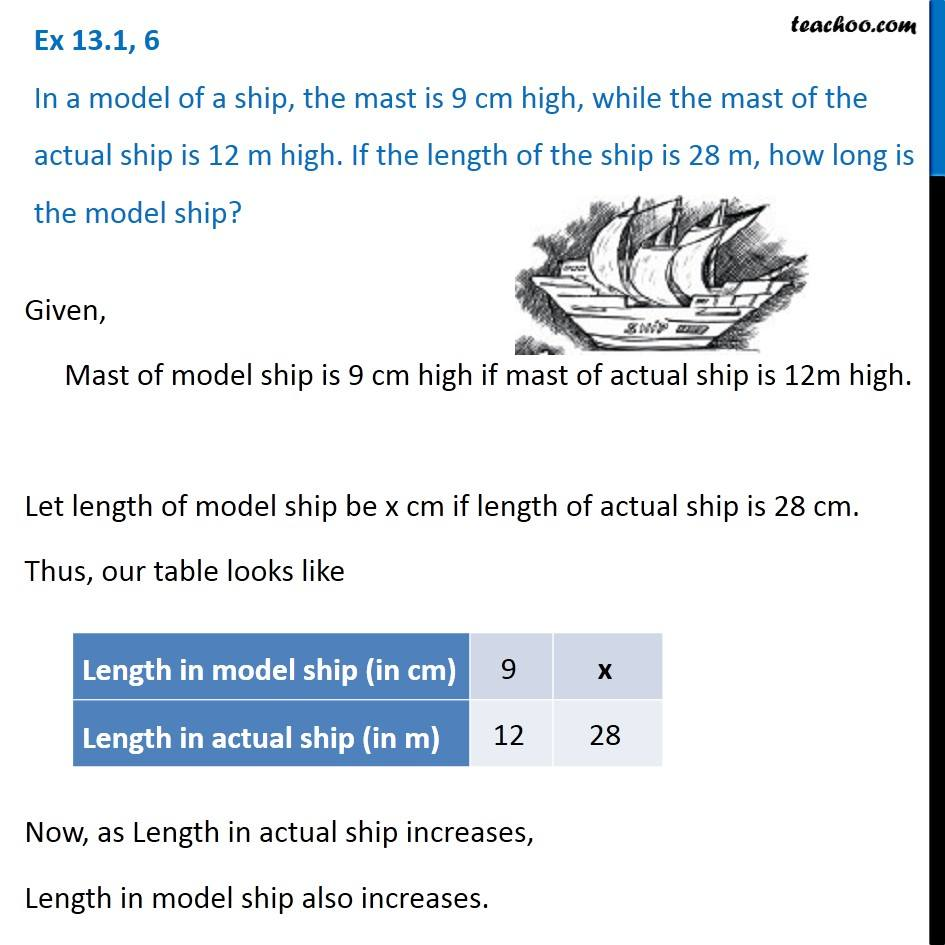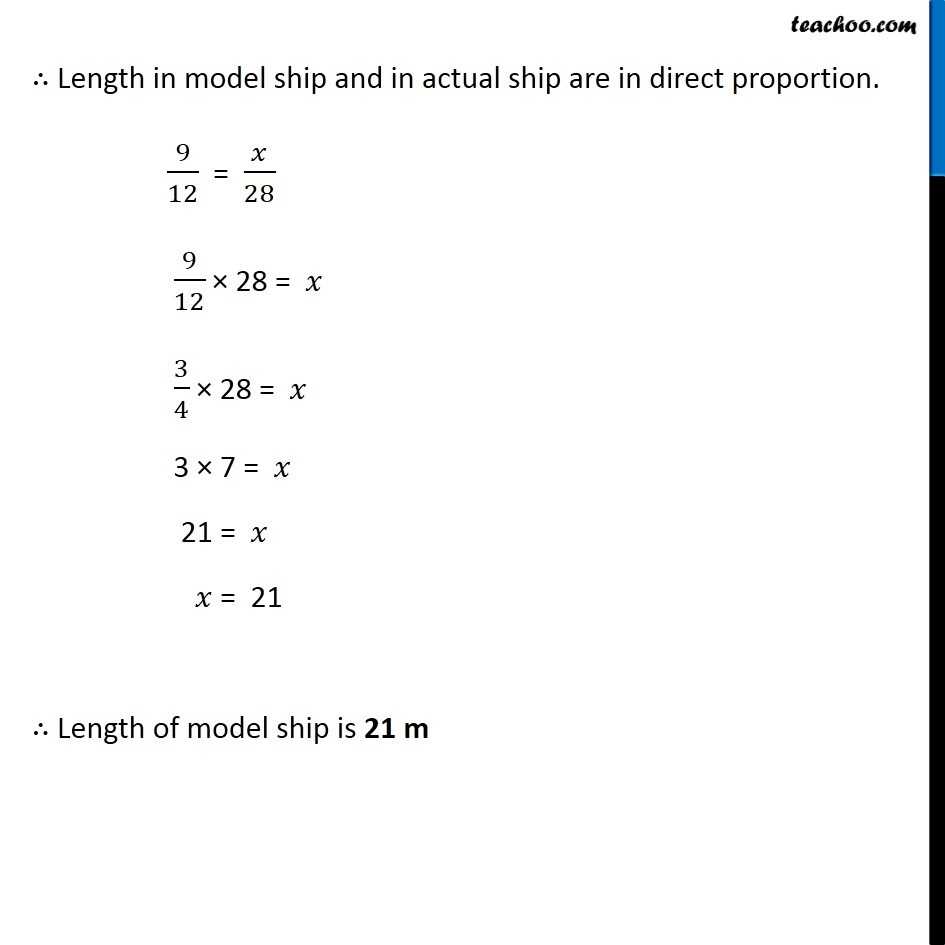1. Chapter 13 Class 8 Direct and Inverse Proportions
2. Concept wise
3. Direct Proportion

Transcript

Ex 13.1, 6 In a model of a ship, the mast is 9 cm high, while the mast of the actual ship is 12 m high. If the length of the ship is 28 m, how long is the model ship? Given, Mast of model ship is 9 cm high if mast of actual ship is 12m high. Let length of model ship be x cm if length of actual ship is 28 cm. Thus, our table looks like Now, as Length in actual ship increases, Length in model ship also increases. ∴ Length in model ship and in actual ship are in direct proportion. 9/12 = 𝑥/28 9/12 × 28 = 𝑥 3/4 × 28 = 𝑥 3 × 7 = 𝑥 21 = 𝑥 𝑥 = 21 ∴ Length of model ship is 21 m